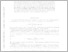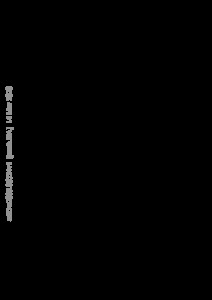Repository: Freie Universität Berlin, Math Department

# Error bounds for PDE-regularized learning

Gräser, C. and Alathur Srinivasan, P. A. (2020) Error bounds for PDE-regularized learning. arXiv:2003.06524 . pp. 1-20. (Submitted)Preview

636kB

Official URL: https://arxiv.org/abs/2003.06524

## Abstract

In this work we consider the regularization of a supervised learning problem by partial differential equations (PDEs) and derive error bounds for the obtained approximation in terms of a PDE error term and a data error term. Assuming that the target function satisfies an unknown PDE, the PDE error term quantifies how well this PDE is approximated by the auxiliary PDE used for regularization. It is shown that this error term decreases if more data is provided. The data error term quantifies the accuracy of the given data. Furthermore, the PDE-regularized learning problem is discretized by generalized Galerkin discretizations solving the associated minimization problem in subsets of the infinite dimensional functions space, which are not necessarily subspaces. For such discretizations an error bound in terms of the PDE error, the data error, and a best approximation error is derived.

Item Type: Article Mathematical and Computer Sciences > Mathematics > Numerical Analysis Department of Mathematics and Computer Science > Institute of Mathematics 2421 Ekaterina Engel 23 Mar 2020 09:27 23 Mar 2020 09:27

Repository Staff Only: item control page